# Gravity – force, energy and accelerationQuestions involving gravitational force and gravitational potential energy are common in the PSLE. Some are questions that even parents struggle to explain. This may be because a lot of content about forces is simplified for the primary school level. Although this makes the concept less intimidating, it becomes more an exercise of rote learning than that of understanding.

Here, I attempt to break the subject down and explain at the primary school level some key concepts. Some examples will be provided at the end to test understanding.

Gravitational Force

A force, as defined in the syllabus, is a “push” or a “pull”. It can

• move a stationary object
• speed up, slow, or change the direction of motion of an object
• stop a moving object
• change the shape of an object.

The first three points addresses the “acceleration” of an object, or the change in speed in the object (speeding up or slowing down or changing direction). Moving a stationary object speeds it up. Stopping an object slows it down.

Gravitational force, sometimes also known as gravity, is a “pull” of an object to the earth. It is a force: it can change the speed and direction of an object. It is always present on all objects, whether it is flying or lying on the ground, or whether it is moving or stationary.

Gravitational force is directly proportional to mass. The greater the mass, the greater the gravitational force. Weight is the measure of the gravitational force between the object and the Earth.  Objects that have the same mass have the same gravitational force.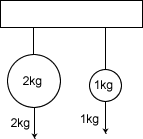The above figure shows that a 2kg mass has a 2kg gravitational force. A 1 kg weight has a 1 kg gravitational force. The greater the mass, the greater the gravitational force.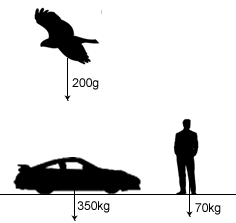The figure above illustrates that gravity is acting on all objects all the time, no matter their speed or orientation. A bird also has to overcome gravity to fly – it will continue to experience a weight of 200g even while flying.

Even a hot air balloon has gravitational force. The reason it floats is because it is less dense than air – not because it is weightless!

Gravitational Potential Energy

Gravitational potential energy is the store of energy of an object due to its position.

The gravitational potential energy is directly proportional to

• its mass
• how high it is above the ground

Example: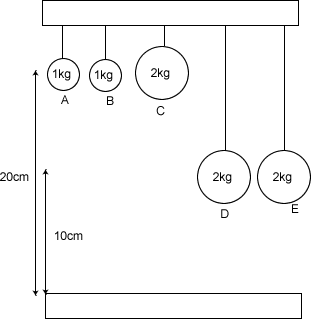A has the same gravitational potential energy as B. D has the same gravitational potential energy as E. C has twice the gravitational potential energy as A and B. C has twice the gravitational potential energy as D and E. B and D have the same gravitational potential energy – this is because B is twice as high up as D but half as heavy.

Gravitational potential energy and gravitational force are different! The gravitational force only depends on the mass of an object. The gravitational potential energy depends on both the mass as well as its position. Gravitational force is the pull on the object, or its weight. Gravitational potential energy is the potential to do work because of its position.

Gravitational acceleration

Gravity speeds up objects by the same amount, regardless of its mass. You can try this experiment – fill two bags with coins: one bag with twice the number of coins as the other. Drop them from the same height. They will hit the ground at the same time.

In the absence of air resistance and other forces, a feather dropped from the same height as an iron ball will also hit the ground at the same time.

Key concepts

• Gravitational force (sometimes known as gravity) is different from gravitational potential energy.
• Gravitational force
• acts on all objects all the time
• for any object it is the same, regardless of its position or speed
• is directly proportional to an object’s mass. The more mass an object has, the more force it experiences
• Gravitational potential energy
• is a store of energy due to the object’s height above the ground
• is directly proportional to an object’s mass.
• Is directly proportional to an object’s height above the ground
• Gravitational force speeds objects up by the same amount, regardless of mass. In the absence of friction and air resistance, all objects will reach the ground at the same time. This is also true for ramps (inclined planes).

Example questions

It’s sometimes better to illustrate the concepts through some example questions.

Q: Two balls are placed on top of a ramp. Ball A has a greater mass than ball B. Which ball reaches the ground first?

A: Both reach the ground at the same time. This is because they both have the same acceleration.

Q: Which of the following objects are not affected by gravitational force?

A. A bird flying in the sky
B. A man lying on the floor
C. A balloon rising into the sky

1. B only
2. A and C only
3. A and B only
4. None of the above

Ans: (4) None of the above. All of the above are affected by gravitational force. (so none of the above are not affected by gravitational force). Even a rising balloon is affected by gravitational force.

Q: “When a ball reaches its max height, the force of gravity acting on the ball is the greatest.”  True or False?

Ans: False. The gravitational force on the ball is the same. The gravitational potential energy is highest when the ball reaches its max height.

Q: The following diagram shows four balls of the same mass hanging from a structure,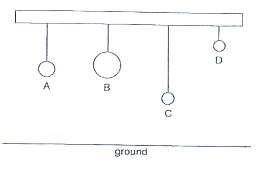Which of the following sentences on the set-up above is true?

(1) all the balls have no gravitational force acting on it.
(2) all the balls have the same gravitational force acting on it.
(3) A has greater gravitational force acting on it as compared to C.
(4) B has greater gravitational force acting on it as compared to D.

Ans: (2).

(1) is false because gravitational force is always present.

(2) is true because all the balls have the same mass (as stated in the qn)

(3) is false. They have the same gravitational force.  A has greater gravitational potential energy because it is higher.

(4) is false because they have the same gravitational force. D has greater gravitational potential energy because it is higher.

Q:  Bob kicked a ball into the air as shown in the diagram below.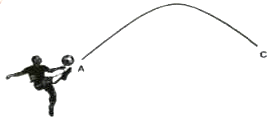Which one of the following graphs shows the gravitational force exerting on the ball when the ball is kicked from position A to C?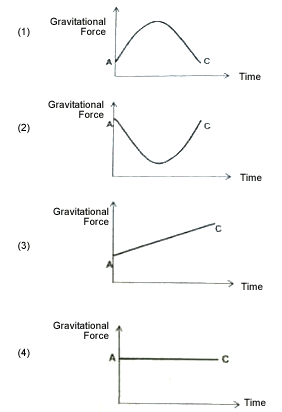Ans: (4)

Gravitational force is the same regardless of position or speed.

Q: The diagram below shows part of a roller coaster ride that can be found in amusement parks.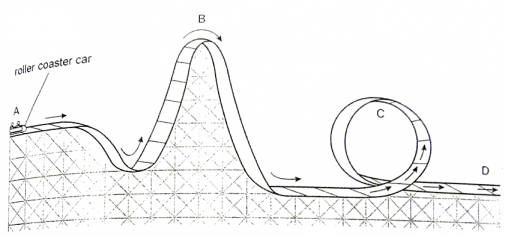The roller coaster car travels from A to D.

Which one of the following statements about the roller coaster car is correct?

(1) Its kinetic energy at C is zero
(2) The gravitational force acting on it at D is zero
(3) The gravitational force acting on it at B is the same as the gravitational force acting on it at A.
(4) Its total potential and kinetic energy at C is greater that its total potential and kinetic energy at B.

Ans: (3) is correct.

(1) Is false because the car is moving.
(2) Is false because gravitational force is always present, regardless of position or speed.
(3) Is correct because gravitational force only depends on mass, and the mass of the roller coaster car is the same
(4) Is false, because the total potential and kinetic energy at C is the same as that in B.

Q: Object X was hung from a spring balance and its mass was measured at 800g. Object Y was hung from the same spring balance, at the same location, and its mass was measured as 500g.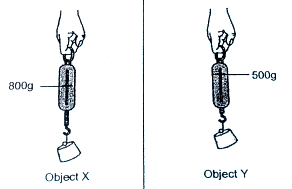Which one of the statements is true about the above observation?

(1) The gravity acting on the objects were the same
(2) The elastic spring force exerted on the objects were the same.
(3) The gravity acting on Object X was stronger than the gravity acting on Object Y.
(4) The elastic spring force exerted on Object X was weaker than the elastic spring force exerted on Object Y.

Ans: (3)
(1) is false. Gravitational force is directly proportional to mass. So X has a higher gravitational force.
(2) is false. Elastic spring force on X is stronger.
(3) is true. Gravity on X is stronger than gravity on Y.
(4) is false. Elastic spring force on X is stronger than elastic spring force on Y.

I hope this helps clarify some of the doubts you may have regarding gravity.

Extra notes:

In secondary school, students learn that the gravitational force between two masses is inversely proportional to the square of the distance between them. This is not necessary to know at the primary school level – it is sufficient to assume that the distance between an object and the center of the Earth is the same wherever the object is on Earth.

Strictly speaking, the kilogram (kg) is a measure of mass, not of weight. Weight is the measure of the gravitational force. However for primary school it is not important to make this distinction yet.

The explanation for why gravitational acceleration is the same regardless of mass comes in secondary school Physics, when students learn the second law of Newton’s laws of motion: F = ma. That is, the change in speed of an object is directly proportional to the force on the object and inversely proportional to its mass. Since the gravitational force that acts on an object is directly proportional to its mass, and the acceleration of the object is proportional to the force and inversely proportional to its mass, it works out that the acceleration of the object due to gravity (also called gravitational acceleration) is independent of mass.